# Conversions Of Units

The conversion of units is extremely crucial for solving some particular topics like number systems, easily and effectively. Also, several direct conversion questions are often asked in the CAT quantitative section which is illustrated below. Learn the conversion techniques of bases and learn various calculations in different bases.

## Concept of Bases and Base Conversion

### Representation in a Particular Base:

Usually, a number N in a particular base x is represented as (N)x

Eg) (1234)6 indicates a number in base 6

As the standard base is base 10, we do not need to show it.

If no base (x) is indicated as a subscript to the number, it is assumed to be in base 10

### Conversion of Base:

#### 1) Base 10 to Other Base Conversion

A two digit number “xy” in base 10 can be expressed as 101x +100y ( the values of x and y will range from 0-9)

If a number is given to you in base 10, you can convert it to the required base by successively dividing it by the number representing the requisite base

For example, conversion of 14 from base 10 to base 2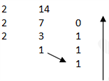( 0 is the remainder obtained when 2 divides 14)

( 1 is the remainder obtained when 2 divides 7)

( 1 is the remainder obtained when 2 divides 3)

( 1 is the remainder obtained when 2 divides 1)

the number 14 in base 2 system will be 1110.

#### 2) Other Base to Base 10 Conversion

If a number is given in another base, say base 2, you can convert it back to base 10 using the following steps.

Let’s take the same example as above.

Convert 1110 in base 2 to base 10

To convert to base 10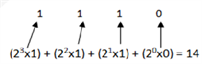### Illustrations:

Question 1: What is 34 in base 2?

Solution: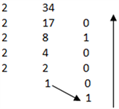Thus 32 in base 2 is 100010.

Question 2: How will you write (1234)6 in base 10?

Solution: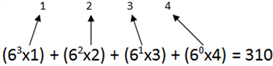Thus (1234)6 is 310 in base 10.

#### 3) Addition, Subtraction and Multiplication in different bases

To add or subtract numbers in a particular base, you can convert the numbers to base 10, perform the operation and then convert the answer back to the requisite base

You can also perform the operation directly by following the steps below

Let us take an example,

4 3 4 5

+ 1 3 2 3

The addition of the units place=5+3 which is 2 more than 6=8

Thus, it can be written as 8=6×1+2.

The remainder will be in the units digit of the answer and the quotient (1) is carried over to the ten’s place

Note that base 6 has digits from 0-5 only 7= 6×1+1

Thus, we get-

+1 +1

4 3 4 5

+ 1 3 2 3

0 1 1 2

Let’s try another example:

1 2 3 4

+ 1 1 5 5

The addition of the units place=5+4 which is 2 more than 7

It can be written as 9=7×1+2.

The remainder will be in the units digit of the answer and the quotient (1) is carried over to the ten’s place

Thus, we get-

+1 +1

1 2 3 4

+ 1 1 5 5

2 4 2 2

• #### SUBTRACTION:

There is a slight difference compared to addition.

Let us understand the method with the help of an example-

Subtract (1234)6 from (4311)6

4 3 1 1

_ 1 2 3 4

Look in the units place. 1 is lesser than 4

We carry a value equal to the base (in this case base=6) from the number on the left.

1+6=7 and 7-4=3. thus we write 3 in the units column.

The 1 in the tens place is now reduced to 0. We carry over the value from the left= the base=6. 6+0=6 and 6-3=3. thus,

we get 3 in the tens place of the answer.

Proceeding this way, the subtraction will yield a result as follows

4 3 1 1

_ 1 2 3 4

3 0 3 3

Thus, in subtraction, the procedure is the same as in base 10, except that when we carry to the right, we carry a value equal to the base in which the question in based

Let us try another example

Subtract (56036)7 from (62342)7

6 2 3 4 2

– 5 6 0 3 6

Look in the units place. 2 is lesser than 6

We carry a value equal to the base (in this case base=7) from the number on the left.

2+7=9 and 9-6=3. Thus we write 3 in the units column.

The 4 in the tens place is now reduced to 3.

in the tens place we will get 3-3=0

Proceeding this way, the subtraction will yield a result as follows

6 2 3 4 2

– 5 6 0 3 6

3 3 0 3. The answer is 3303.

• #### MULTIPLICATION:

Let us look at multiplication in base 8, for example-

(57)8 x (7)8

57 x 7

7×7 =49 = 8×6 +1 ( We write 1 and carry 6)

(7×5)=35+6(carry)=41 = 8×5 +1 ( we write 1 and carry is 5)

Let us try another method, where we convert all numbers back to base 10, do the multiplication; and then convert it back to base 8

(57)8 can be written in base 10 as?7x(1)+5x(8)=47

(7)7 is 7 itself

(47)x7 = 329

Write 329 in base 8Hence, the answer is 511. you can choose whichever method is easier for you.

### Illustrations:

1. (34)7 x (6)7

Solution:

Using the first method 34 x6 In the units place? 4×6=24 24 can be written as 24=7×3+3 (we write 3 and carry 3)Similarly 6×3=18.

Add 18 to 3(Carry)= 21 21 can be written as 21=7×3+0( we write 0 and carry 3)

Thus the answer is (303)7 11).

2. What is (127)8 – (12)6 = (?)5

a) 230

b) 124

c) 340

d) none of these

Solution:

Write all the numbers in base 10 and then arrive at the answer 127 in base 8 = 7×80 + 2×81 + 1×82= 87 in base 10 12 in base 6 = 2×60+ 1×61= 8 in base 10

Adding both = 95 in base 10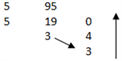Expressing in base 5=340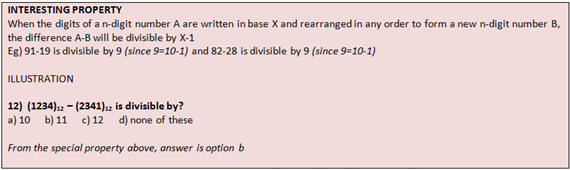Stay tuned with Byju’s to get more such lessons on important CAT exam topics along with various engaging video lectures to prepare more effectively for the exam. Also, get latest notifications, syllabus and numerous sample papers to get acquainted with the CAT paper accurately.